﻿ NLVM Pre-K - 2 Manipulatives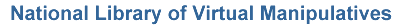All Topics (Grades Pre-K - 2)

Virtual manipulatives for grades Pre-K - 2.

Number & Operations (Grades Pre-K - 2)Bar Chart – Create a bar chart showing quantities or percentages by labeling columns and clicking on values.Base Blocks – Illustrate addition and subtraction in a variety of bases.Base Blocks Addition – Use base ten blocks to model grouping in addition.Base Blocks Decimals – Add and subtract decimal values using base blocks.Base Blocks Subtraction – Use base ten blocks to model separation of groups in subtraction.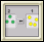Chip Abacus – Learn about carrying and digits using chips.Circle 99 – A puzzle involving adding positive and negative integers to sum to ninety nine.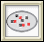Color Chips - Addition – Use color chips to illustrate addition of integers.Color Patterns – Arrange colors to complete a pattern.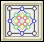Diffy – Solve an interesting puzzle involving the differences of given numbers.Fraction Bars – Learn about fractions using fraction bars.Fractions - Naming – Write the fraction corresponding to the highlighted portion of a shape.Fractions - Parts of a Whole – Relates parts of a whole unit to written description and fraction.Fractions - Visualizing – Illustrate a fraction by dividing a shape and highlighting the appropriate parts.Hundreds Chart – Practice counting and visualize number patterns using a hundreds chart.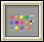Mastermind – Use inference and logic to play a game and guess a hidden pattern of pegs.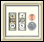Money – Learn about money by counting and making change.Number Line Arithmetic – Illustrates arithmetic operations using a number line.Number Line Bars – Use bars to show addition, subtraction, multiplication, and division on a number line.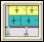Number Line Bars - Fractions – Divide fractions using number line bars.Number Line Bounce – Number line addition and subtraction game.Number Patterns – Discover the pattern and complete a sequence of numbers.Percentages – Discover relationships between fractions, percents, and decimals.Pie Chart – Explore percentages and fractions using pie charts.Place Value Number Line – Explore place value by placing dots on number lines.Rectangle Division – Visualize and practice dividing numbers by using an area representation.Rectangle Multiplication – Visualize the multiplication of two numbers as an area.Rectangle Multiplication of Integers – Visualize and practice multiplying integers using an area representation.Sieve of Eratosthenes – Relate number patterns with visual patterns.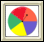Spinners – Work with spinners to learn about numbers and probabilities.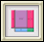Algebra Tiles – Visualize multiplying and factoring algebraic expressions using tiles.Color Patterns – Arrange colors to complete a pattern.Fifteen Puzzle – Solve this virtual version of the classical fifteen puzzle by arranging its tiles.Pattern Blocks – Use six common geometric shapes to build patterns and solve problems.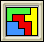Pentominoes – Use the 12 pentomino combinations to solve problems.Polyominoes – Build and compare characteristics of biominoes, triominoes, quadrominoes, etc.Triominoes – Manipulate the puzzle pieces and find multiple solutions.Turtle Rectangles – Change variables to observe differences in spiral designs.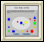Attribute Blocks – Learn color and shape concepts by sorting blocks.Attribute Trains – Learn about shape and color patterns of by completing trains of blocks.Congruent Triangles – Build similar triangles by combining sides and angles.Geoboard – Use geoboards to illustrate area, perimeter, and rational number concepts.Geoboard - Isometric – Use geoboard to illustrate three-dimensional shapes.Ladybug Leaf – Program a ladybug to hide behind a leaf.Ladybug Mazes – Program a ladybug to move through a maze.Pattern Blocks – Use six common geometric shapes to build patterns and solve problems.Pentominoes – Use the 12 pentomino combinations to solve problems.Platonic Solids – Identify characteristics of the Platonic Solids.Space Blocks – Create and discover patterns using three dimensional blocks.Tangrams – Use all seven Chinese puzzle pieces to make shapes and solve problems.Tessellations – Using regular and semi-regular tessellations to tile the plane.Transformations - Reflection – Dynamically interact with and see the result of a reflection transformation.Transformations - Rotation – Dynamically interact with and see the result of a rotation transformation.Transformations - Translation – Dynamically interact with and see the result of a translation transformation.Triominoes – Manipulate the puzzle pieces and find multiple solutions.Turtle Geometry – Explore numbers, shapes, and logic by programming a turtle to move.Attribute Blocks – Learn color and shape concepts by sorting blocks.Attribute Trains – Learn about shape and color patterns of by completing trains of blocks.Geoboard – Use geoboards to illustrate area, perimeter, and rational number concepts.Ladybug Leaf – Program a ladybug to hide behind a leaf.Ladybug Mazes – Program a ladybug to move through a maze.Money – Learn about money by counting and making change.Pattern Blocks – Use six common geometric shapes to build patterns and solve problems.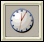Time - Analog and Digital Clocks – Interactively set the time on a digital and analog clocks.Time - Match Clocks – Answer questions asking you to show a given time on digital and analog clocks.Time - What Time Will It Be? – Answer questions asking you to indicate what time it will be before or after a given time period.Bar Chart – Create a bar chart showing quantities or percentages by labeling columns and clicking on values.Pie Chart – Explore percentages and fractions using pie charts.Spinners – Work with spinners to learn about numbers and probabilities.